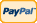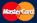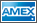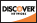Online Store Software Services News About Us My Account Español
•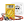I need to measure...
•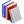I need to calculate...
•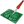Common electronic components repairs and hand soldering techniques
•Electronics, electrical engineering
•Engineering physics
•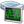Generators
•History events
•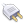Interfaces
•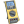Multimeters
•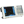Oscilloscopes
•Persona
•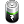Power supplies
•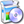Software
•Standards
•Thesaurus
•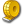Units of measurement

Filter by first letter

# Joule (J)

The joule, symbol J, is a derived unit of energy, work, or amount of heat in the International System of Units. It is equal to the energy expended (or work done) in applying a force of one newton through a distance of one metre (1 newton meter or N·m), or in passing an electric current of one ampere through a resistance of one ohm for one second. It is named after the English physicist James Prescott Joule.

In terms firstly of base SI units and then in terms of other SI units: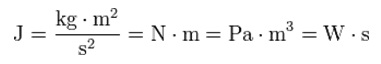where N is the newton, m is the meter, kg is the kilogram, s is the second, Pa is the pascal, and W is the watt.

One joule can also be defined as:

• The work required to move an electric charge of one coulomb through an electrical potential difference of one volt, or one '"coulomb volt" (C·V). This relationship can be used to define the volt.
• The work required to produce one watt of power for one second, or one "watt second" (W·s) (compare kilowatt hour). This relationship can be used to define the watt.

www.wikipedia.org

Back to the list

Rating

Measurement History Events
 Yesterday Today Tomorrow
09/25/1928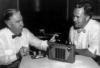09/25/1926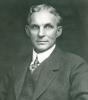09/25/1820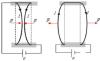Units Converter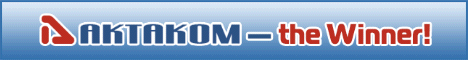Site map|Privacy policy|Terms of Use & Store Policies|How to Buy|Shipping|Payment|© T&M Atlantic, Inc., 2010-2023